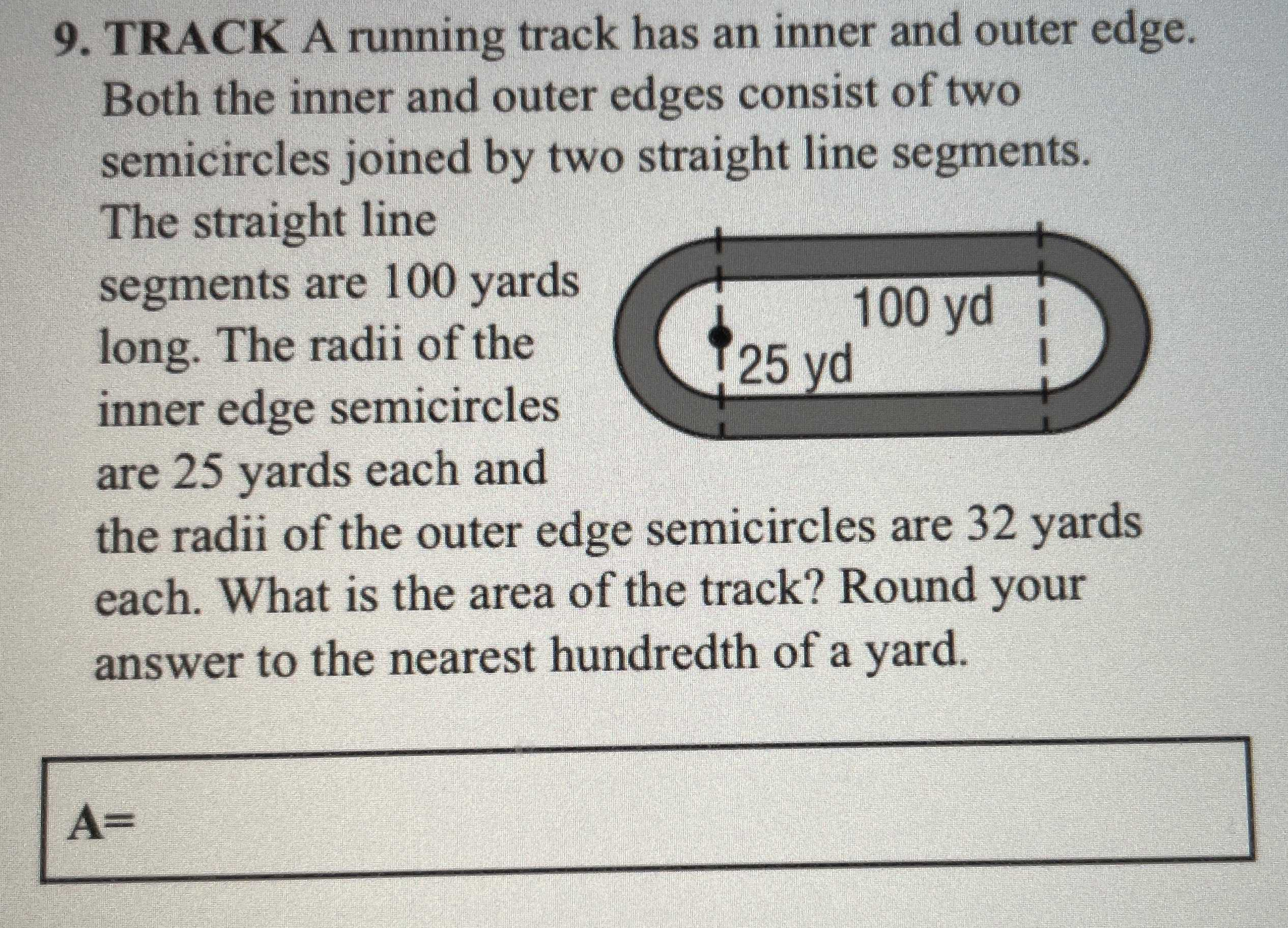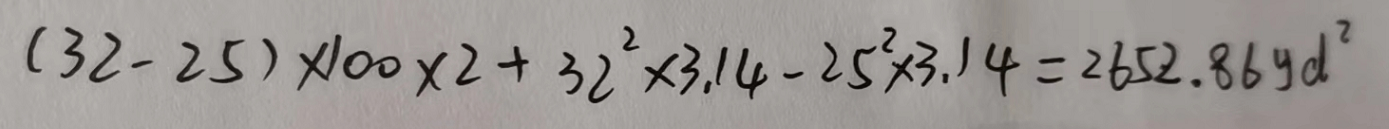### ¿Todavía tienes preguntas de matemáticas?

Pregunte a nuestros tutores expertos
Algebra
Pregunta9. TRACK A running track has an inner and outer edge. Both the inner and outer edges consist of two semicircles joined by two straight line segments. The straight line segments are $$100$$ yards long. The radii of the inner edge semicircles are $$25$$ yards each and the radii of the outer edge semicircles are $$32$$ yards each. What is the area of the track? Round your answer to the nearest hundredth of a yard.

$$A =$$